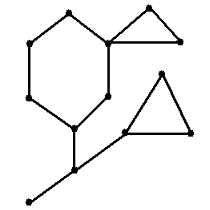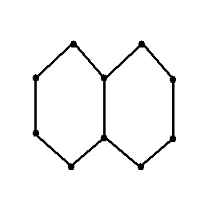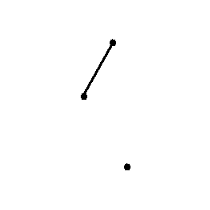### Cactus

A cactus graph is a simple, undirected, and connected graph in which every edge belongs to at most one simple cycle. A simple graph is a graph with no self-edges or multi-edges, while a simple cycle is a cycle which contains no repeated nodes.A cactus graph.Not a cactus graph - an edge is contained in two simple cycles.Not a cactus graph - the graph is not connected. Jiutiao has a simple, undirected, and connected graph with $$n$$ vertices numbered from $$1$$ to $$n$$, and $$m$$ edges. However, she feels that this graph has too few edges, and wants to add some new edges onto its vertices. At the same time, in order to keep her graph convenient to store, Jiutiao should not make the graph too dense. Weighing her options, Jiutiao decided that she would add edges to her graph such that it ends up as a cactus.

It is simple to see that there may be many different ways to add edges to the graph. Jiutiao would like to know how many schemes there are in which edges are added to turn the graph into a cactus.

Two schemes are considered distinct if and only if there is an edge which is added in one scheme, but not the other.

### Input format

The first line of input consists of a single integer $$T$$, the number of testcases. $$T$$ testcases follow.
The first line of each testcase consists of two integers $$n$$ and $$m$$, denoting the number of nodes and number of edges in Jiutiao's graph respectively.
$$m$$ lines follow. The $$i^{th}$$ of these lines contains 2 integers $$u_i$$ and $$v_i$$, denoting that the graph contains an edge between vertices $$u_i$$ and $$v_i$$. It is guaranteed that there will be no self-edges or multi-edges, and the graph is connected.

### Output format

Output a single line for each testcase, containing a single integer - the number of schemes adding edges such that the graph ends up a cactus.
As this number may be very large, output its value modulo $$998244353$$.

### Limits

$$1 \leq T \leq 10^4$$.
$$1 \leq n \leq \sum{n} \leq 5 \times 10^5$$.
$$1 \leq u_i, v_i \leq m \leq \frac{n(n - 1)}{2}$$.
$$1 \leq \sum{m} \leq 10^6$$.

1 10 $$n \leq 5$$
$$m \leq 10$$
2 20 $$n \leq 2000$$
$$m \leq 2 \times 10^5$$
3 20 $$n \leq 10^5$$
$$m = n - 1$$
The graph is a line.
4 20 $$n \leq 10^5$$
$$m = n - 1$$

### Samples

 Sample Input 1 Sample Output 1 2 3 2 1 2 1 3 5 4 1 2 2 3 2 4 1 5 28

In testcase 1, there are two valid schemes for adding edges: $$\{\}$$ and $$\{(2, 3)\}$$.
In testcase 2, there are eight valid schemes for adding edges: $$\{\}$$, $$\{(1, 3)\}$$, $$\{(1, 4)\}$$, $$\{(2, 5)\}$$, $$\{(3, 5)\}$$, $$\{(3, 4)\}$$, $$\{(4, 5)\}$$, and $$\{(2, 5), (3, 4)\}$$.

### Submitting .cpp to 'cactus'

Time Limit: 1.5 Seconds
Memory Limit: 1024MB